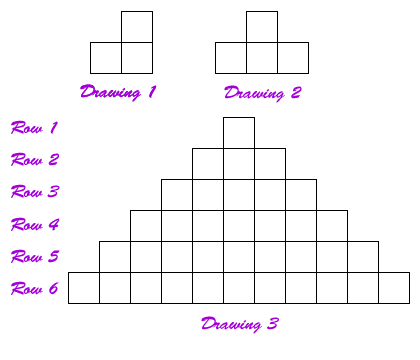# Stairways and Blocks

## The problem

A student diagrammed a stairway using squares to represent blocks that could be used to build stairways of different heights. After the first drawing the student though it would be better if the staircase went up and down. Therefore, drew the second drawing. Satisfied that was better continued with the pattern and drew the third drawing.1. Each row is made with blocks. How many blocks are in each row?
• Row 1
• Row 2
• Row 3
• Row 4
• Row 5
• Row 6
• If the staircase were made higher, how many blocks would be needed for row 8?
• Row 9?
• How about row 15?
• Write a rule you could use to determine the number of blocks needed for row 20 and row 50?
• Write a rule you could use to determine how many blocks would be in any row in the pattern (for example: row #n).
1. Each complete stair case is made with a certain number of blocks. How many blocks are needed to build the staircase in drawing 3?
• If the staircase were made one block higher, how many blocks would be needed to make that 7 row/step-up staircase?
• How many blocks would be needed for a 9 row/ step-up staircase?
• How about 15?
• Write a rule you could use to determine the number of blocks needed for a 20 step-up staircase and 30 step-up staircase?
• Write a rule you could use to determine how many blocks would be need to build a staircase if the number of steps-up the staircase was known. (for example: step-up #n).
1. If the number of blocks in the bottom row was known, how could the number of blocks needed to build a double sided staircase be determined?
• If the bottom row had 39 blocks, How many blocks would be in the double sided staircase?
• If the number of blocks in the bottom row was known, how could the number of blocks needed to make a double sided staircase, of this type, be determined?